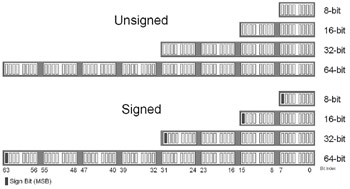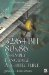Processor OverviewThere are a large variety of computers with different processors and different word sizes but there is one constant, the byte. Memory in a computer is represented as a series of bytes and each of these bytes is made up of eight bits. This allows an unsigned value ranging from 0255 or a signed value ranging from 1280127 to be stored in each byte. These eight bits can store one ASCII character AZ. These bytes can be used together to form larger data structures such as a 16-bit short, 32-bit int, 64-bit long, etc.

In a higher level language such as C this is typically represented by a hex value. For example, 123 decimal is: 64+32+16+8+2+1.

2 7
128

2 6
64

2 5
32

2 4
16

2 3
8

2 2
4

2 1
2

2
1

1

1

1

1

1

1

So binary 01111011 broken into nibbles (4-bit chunks ) 0111 1011 is 7B hex. I did it for you here, but you really should already know how to do decimal-to-hex and hex-to-decimal conversions. In the C programming language this is represented by 0x7B. In an assembler such as MASM this can be represented in a variety of ways:

mov eax, 123        ; Decimal mov eax, 7bh        ; Hex mov eax, 01111011b  ; Binary

Let's try that again but with a slightly bigger number in which the most significant bit (MSB) gets set.

2 7
128

2 6
64

2 5
32

2 4
16

2 3
8

2 2
4

2 1
2

2
1

1

1

1

1

This maps to 1010 0101 = 0a5h.

It should be pointed out that a number represented in hex in C only needs a leading 0x to indicate that the trailing digits are hex code. In assembly language the suffix of h indicates the value is hex. But if the first digit is not a digit but an alpha value of AF, then a leading zero is required. Therefore, a hex value in assembly language must always begin with a digit even if it is zero. Letters indicate the word about to be processed by the assembler is a label and not a value! Hex letters AF can be mixed and matched upper- and/or lowercase; capitalization does not matter.

We are using the value of 0a5h = 1 0100101B. The B represents binary and the MSB indicated in bold is a 1. If this were an unsigned value ranging from 0255, then 0a5h would resolve to 128+32+4+1 = 165 decimal. Numbers without prefixes or suffixes are in decimal. But what if this were a negative number? 0a5h is a decimal value of 91. How did we do that? Well, we needed something called a two's complement. This is a one's complement followed by an addition of +1.

Since the MSB is set and this is a signed number ranging from 128 to 127, then first NOT (meaning flip) all the bits in the number.

2 7
128

2 6
64

2 5
32

2 4
16

2 3
8

2 2
4

2 1
2

2
1

1

1

1

1

1

1

1

1

The bit sequence of 0101 1010 gives us 5Ah. (Just a coincidence ; I chose the 5 and A on purpose since they are complements of each other! Now add 1 to that: 5Ah+1=5Bh= 01011011B = 64+16+8+2+1 = 91. Since we performed the two's complement we also stick the negative sign () back on it: (91), thus 91. Again, this should be review for you but I want to make sure you understand signed versus unsigned values and how to handle one or the other.

 Note To help alleviate any confusion between this book and my vector book, this one was written for multiple processors. Both books share a generic calling convention and a standard naming convention for data types: (b)yte 8-bit, (h)alf-word 16-bit, (w)ord 32-bit,(d)word 64-bit, and (q)word 128-bit. They are used for function declarations to maintain compatibility between books as well as processor types. Even though the 80x86 does not use a half-word declaration, I forced it to do so for easier understanding of the vector book. This book is strictly about 80x86 assembly language, and its letter encoding is directly connected to data types and instructions, so the specific 80x86 convention will be used here: (b)yte 8-bit, (w)ord 16-bit,(d)word 32-bit, (q)word 64-bit, and (dq)word 128-bit. Please keep this in mind if you are switching back and forth between the two books!
 History The declaration of "word" has a bit of a history. When the 8086 processor was first produced it had 16-bit registers. At that time a word was considered the width of the data, so a word became the definition for a 16-bit value. Other processors had a data width of 32 bits, so a word was used to represent 32 bits. With the release of the 80386, the word was already embedded in the 80x86 assembly code to represent 16 bits, and all code would have to be modified accordingly , so 32 bits came to be known as double words. And so a schism of bit widths related to the definition of a word came to be. In high- level languages such as C an integer (int) was used to represent a word. Since it was not directly tied to an absolute data width, it expanded with time. With the 8086 an int and a short were 16 bits, but with 32-bit processors the int came to represent 32-bit data, while the short still represents 16-bit data.
Table 3-1: 80x86 data types

C type

procType

Bytes

Bits

char

b

byte

1

8

short

w

word

2

16

int

d

dword

4

32

long

q

qword

8

64

SSE, SSE2 (128-bit)

long long

dq

dqword

16

128Figure 3-1: Unsigned/signed data types32/64-Bit 80x86 Assembly Language Architecture
ISBN: 1598220020
EAN: 2147483647
Year: 2003
Pages: 191

Similar book on Amazon